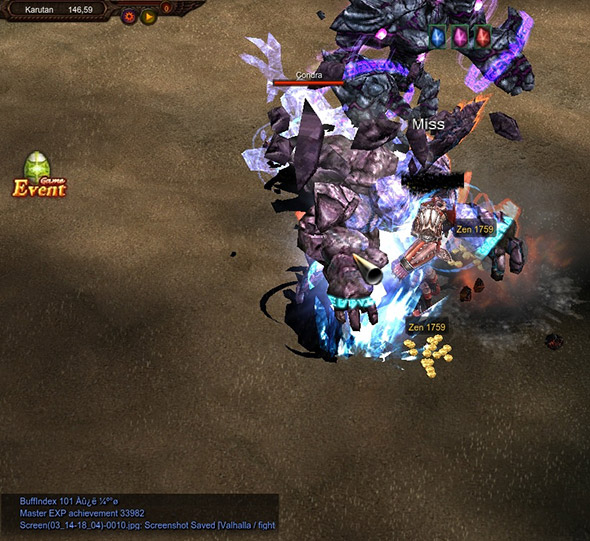## Base Exp x (Channel + Exp Event) x (1 + Ascension + Wealthy + Healing + Pet + Ring + Bonus Exp) x (1 + Premium Exp Bonus)

1) Base Exp : EXP gained while playing in free servers without any addition � Depends on the monster

2)
Exp Event : EXP rate increased due to event - Depends on the current event

3)
Channel - EXP rate increased while playing in free or gold Channel.

100% in free servers
333% for normal levels in gold servers
200% for master levels in gold servers

4) 1 (current EXP) - EXP gained before activating seals, pets, rings or premium exp bonus

Can be understood as the EXP that will be used to calculate the experience boost
Seal - EXP rate increased while using Seal
50% EXP rate increase for Seal of Ascension (Premium EXP Bonus: 10%)
50% EXP rate increase for Master Seal of Ascension (Premium EXP Bonus: 10%)
100% EXP rate increase for Seal of Wealth (Premium EXP Bonus: 20%)
100% EXP rate increase for Master Seal of Wealth (Premium EXP Bonus: 20%)
50% EXP rate increase for Seal of Healing (Premium EXP Bonus: 10%)

Pet - EXP rate increased while using pet (Panda, Skeleton)
50% EXP rate increase for Panda Pet (Premium EXP Bonus: 10%)
50% EXP rate increase for Skeleton Pet (Premium EXP Bonus: 10%)
Ring - EXP increased while using ring (Brown Panda Ring, Pink Panda Ring)
30% EXP rate increase for Brown Panda Ring (Premium EXP Bonus: 10%)
50% EXP rate increase for Pink Panda Ring (Premium EXP Bonus: 10%)
All of Transformation rings with Pet can get bonus EXP 30%
Bonus EXP - EXP increased while using Panda or Skeleton pet with transformation rings
30~65% EXP rate increase depending on the combination

5) Premium EXP Bonus - EXP rate increased while using any shop item that increases EXP.

[EXP increase rate Example]

[Normal  Level / Base EXP 808]

Normal Channel + EXP event (30%)

(1+0.3) = 1.3 > about 1050 EXPNormal Channel + EXP event (30%)+Seal of Healing

(1+0.3) x (1+0.5) x (1+0.1) = 2.14 > about 1732 EXPNormal Channel + EXP event (30%)+Seal of Ascension

(1+0.3) x (1+0.5) x (1+0.1) = 2.14 > about 1730 EXPNormal Channel + EXP event (30%)+Seal of Wealth

(1+0.3) x (1+1.0) x (1+0.2) = 3.12 > about 2520 EXPNormal Channel + EXP event (30%)+Panda pet

(1+0.3) x (1+0.5) x (1+0.1) = 2.14 > about 1730 EXPNormal Channel + EXP event (30%)+ Seal of Ascension + Healing + Wealth

(1+0.3) x (1+0.5+0.5+1.0) x (1+0.1+0.1+0.2)=5.46 > about 4410 EXP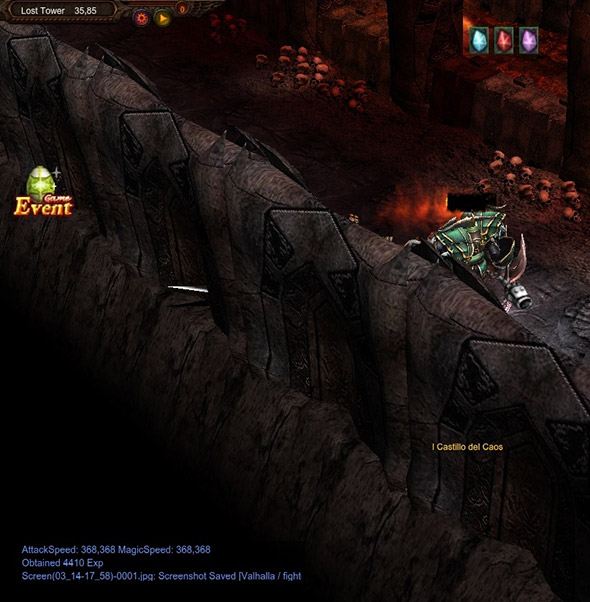Normal Level + Gold Channel + EXP event (30%)

(1+2.33 + 0.3) = 3.63 > about 2933 EXPNormal Level + Gold Channel + EXP event (30%) + Seal of Ascension

(1+2.33 + 0.3) x (1+0.5) x (1+0.1) = 5.98 > about 4839 EXP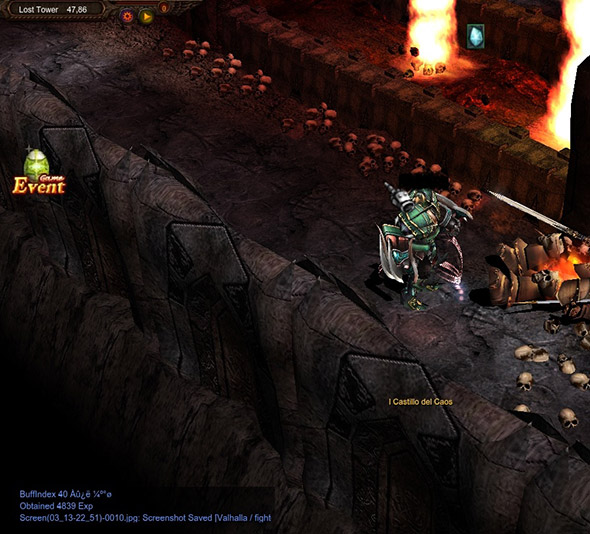Normal Level + Gold Channel + EXP event (30%) + Seal of Healing

(1+2.33 + 0.3) x (1+0.5) x (1+0.1) = 5.98 > about 4839 EXP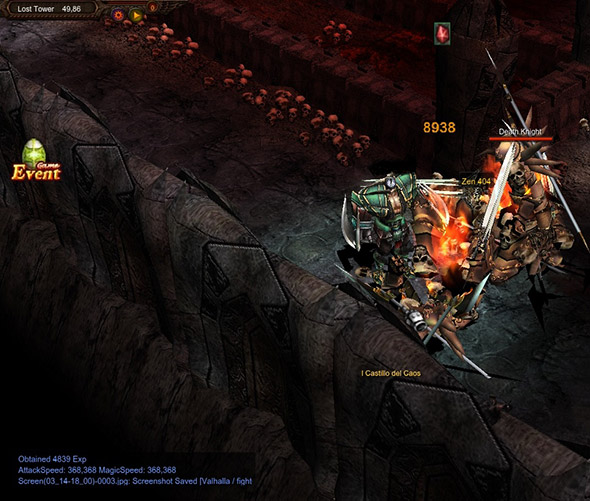Normal Level + Gold Channel + EXP event (30%) + Seal of Wealth

(1+2.33 + 0.3) x (1+1.0) x (1+0.2) = 8.71 > about 7039 EXP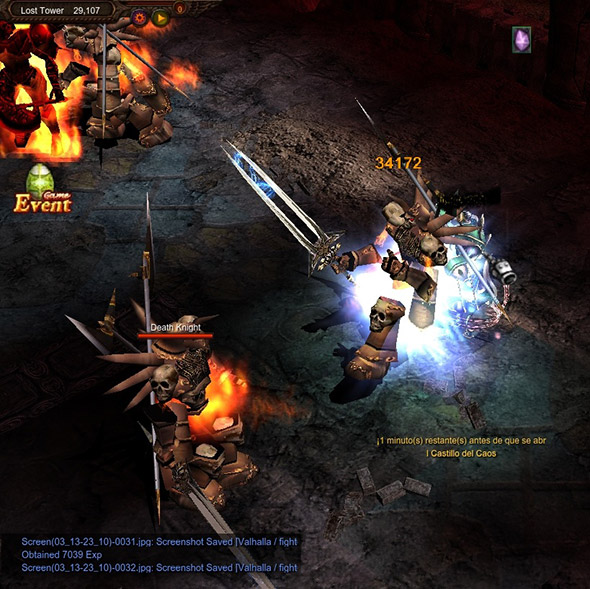Normal Level + Gold Channel + EXP event (30%) + Pet Panda

(1+2.33 + 0.3) x (1+0.5) x (1+0.1) = 5.98 > about 4839 EXP[Master Level / Normal Channel / Base EXP 3518 ]

Master Level + Normal Channel + EXP event (30%)

(1+0.3) = 1.3 > about 4573 EXPMaster Level + Normal Channel + EXP event (30%) + Seal of Healing

(1+0.3) = 1.3 > about 4573 EXP (Seal of healing must be used with other master seal)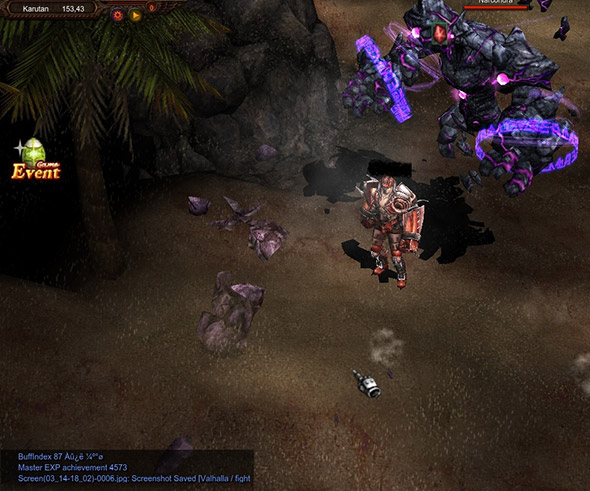Master Level + Normal Channel + EXP event (30%) + Master Seal of Wealth

(1+ 0.3) x (1+ 1.0) x (1+ 0.2) = 3.12 > about 10975 EXP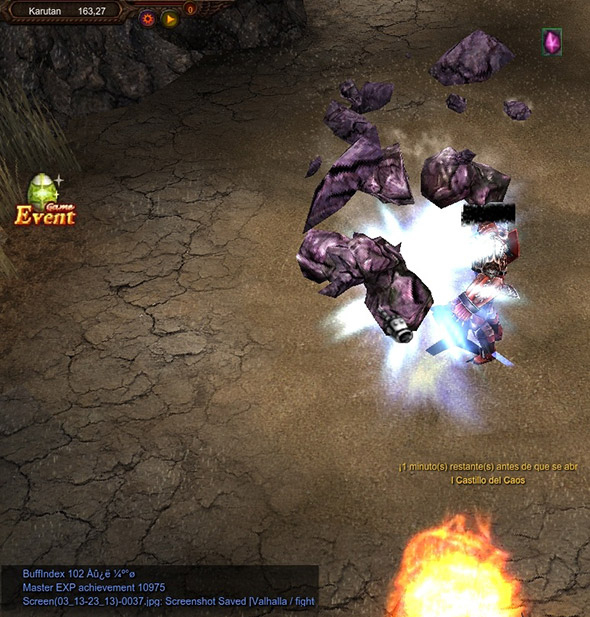Master Level + Normal Channel + EXP event (30%) + Master Seal of Ascension

(1+ 0.3) x (1+ 0.5) x (1+ 0.1) = 2.14 > about 7545 EXP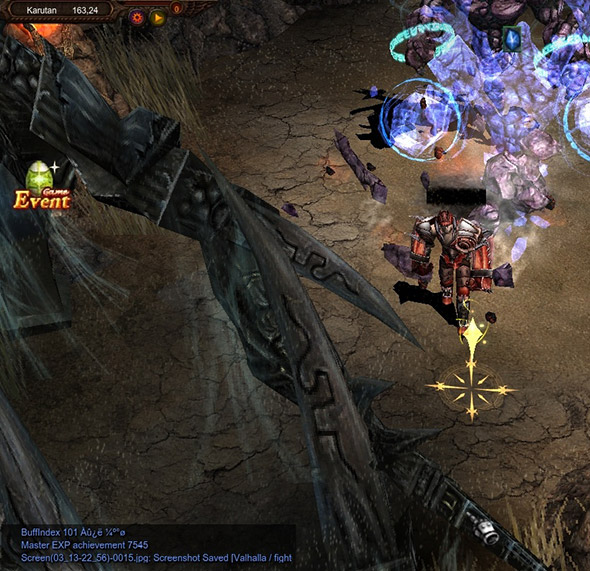Master Level + Normal Channel + EXP event (30%) + Seal of Healing + Wealth(Master)+ Ascension(Master)

(1+ 0.3) x (1+0.5+0.5+1.0) x (1+0.1+0.1+ 0.2) = 5.46 > about 19206 EXP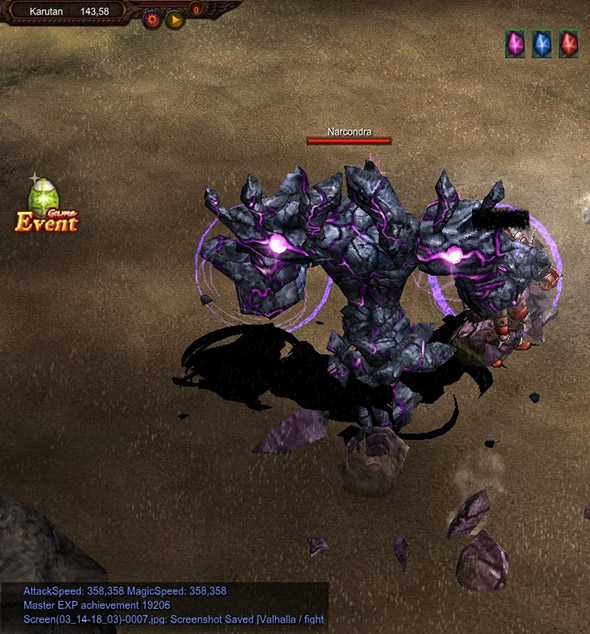[Master Level / Gold Channel / Base EXP 3517]

Master Level + Gold Channel + EXP event (30%)

(2.0+0.3) = 2.3 > about 8091 EXPMaster Level + Gold Channel + EXP event (30%) + Master Seal of Wealth

(2.0+0.3) x (1+ 1.0) x (1+ 0.2) = 5.52 > about 19418 EXPMaster Level + Gold Channel + EXP event (30%) + Master Seal of Ascension

(2.0+0.3) x (1+ 0.5) x (1+ 0.1) = 3.79 > about 13350 EXPMaster Level + Gold Channel + EXP event (30%) + Master Seal of Ascension + Panda pet + Skeleton Ring

(2.0+0.3) x (1+0.5+0.5+0.5) x (1+0.1+0.1+0.1) = 7.47 > about 26295 EXP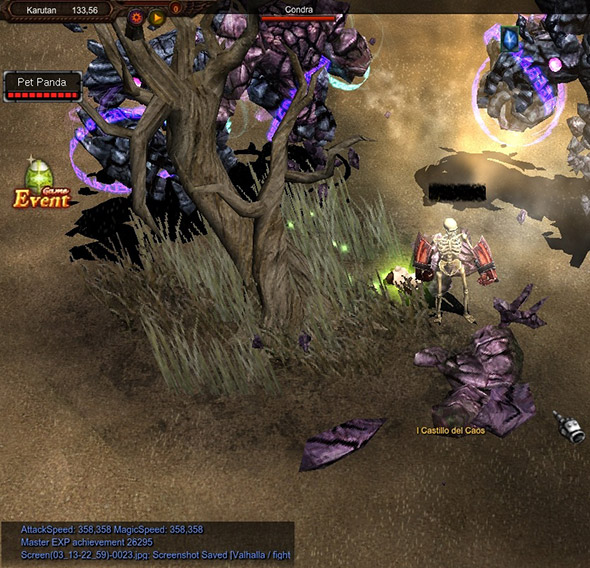Master Level + Gold Channel + EXP event (30%) + Master Seal of Ascension + Panda pet + Skeleton Ring

(2.0+0.3) x (1+1.0+0.5+0.5) x (1+0.2+0.1+0.1) = 9.66 > about 33982 EXP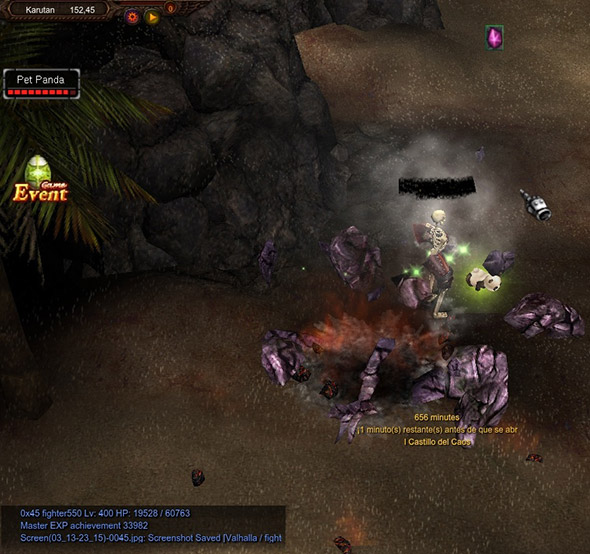Master Level + Gold Channel + EXP event (30%) + Seal of Healing + Ascension(Master) + Wealth(Master)

(2.0+ 0.3) x (1+0.5+0.5+ 1.0 ) x (1+0.1+0.1+0.2) = 9.66 > about 33982 EXP